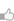求知 文章 文库 Lib 视频 iPerson 课程 认证 咨询 工具 讲座 Model Center Code

 Tcl 教程 Tcl环境设置 Tcl特殊变量 Tcl基本语法 Tcl命令 Tcl数据类型 Tcl变量 Tcl运算符 Tcl决策 Tcl循环 Tcl数组 Tcl字符串 Tcl列表 Tcl字典 Tcl过程 Tcl包 Tcl命名空间 Tcl文件I/O Tcl错误处理 Tcl内置函数 Tcl正则表达式
TCL过程
 667 次浏览31次捐助

 ```proc procedureName {arguments} {body}```

 ```#!/usr/bin/tclsh proc helloWorld {} {puts "Hello, World!"}helloWorld```

 `Hello, World!`

 ```#!/usr/bin/tclsh proc add {a b} {return [expr \$a+\$b]}puts [add 10 30]```

 `40`

 ```#!/usr/bin/tclsh proc avg {numbers} {set sum 0foreach number \$numbers {set sum [expr \$sum + \$number]}set average [expr \$sum/[llength \$numbers]] return \$average}puts [avg {70 80 50 60}]puts [avg {70 80 50 }]```

 `6566`

 ```#!/usr/bin/tclsh proc add {a {b 100} } {return [expr \$a+\$b]}puts [add 10 30]puts [add 10]```

 `40110`

 ```#!/usr/bin/tclsh proc factorial {number} {if {\$number <= 1} {return 1} return [expr \$number * [factorial [expr \$number - 1]]] }puts [factorial 3]puts [factorial 5]```

 `6120`
 您可以捐助，支持我们的公益事业。 1元 10元 50元 认证码：必填
 667 次浏览31次捐助